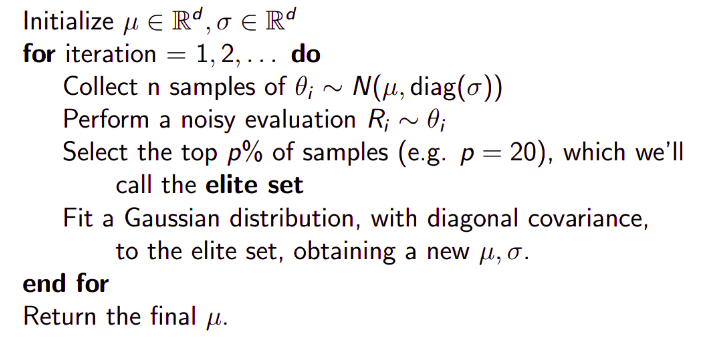If you have ever gathered the courage to explore the field of Reinforcement Learning, there are good chances that you found yourself lost in fancy vocabulary. Big words, names of complex algorithms with even more complex math behind them. But what if there were simpler, much more intuitive, and super-efficient algorithms out there that worked well?

Meet the Cross-Entropy Method: An evolutionary algorithm for parameterized policy optimization that John Schulman claims works “embarrassingly well” on complex RL problems².

##### What is Cross-Entropy Method?

From a biological viewpoint, it is an Evolutionary Algorithm. Some individuals are sampled from a population and only the best ones govern the characteristics of future generations.

Mathematically, it can be seen as a Derivative-Free Optimization (DFO) technique, i.e., it can find optima without the overhead of calculating derivatives (no backpropagation!).

##### How does this method work?

Assume for a second that you do not know what are agents, environments, and policies. You are just given a “black-box” which takes some numbers as inputs and outputs some other numbers. You can only choose the values for your inputs and observe the outputs. How do you guess the inputs such that the outputs are the values you want?

One simple way of doing this would be to take a bunch of inputs, see the outputs produced, choose the inputs that have led to the best outputs and tune them till you are satisfied with the outputs you see. This is essentially what the cross-entropy method does.

##### So, how do I use it to solve my RL problem?

Let’s understand the working of CEM step-by-step with an example. I have added some python code snippets with each step for a better understanding of the implementation. The code is heavily borrowed from Udacity’s course on Deep Reinforcement Learning (amazing python RL resources btw, Github link at the end of this article)¹.

Consider your policy network. You want to find the best weights which can take the right “meaningful” actions based on your agent’s state. A CEM-based approach for finding these weights is as follows:

Step 1: Draw a bunch of initial weights from a random distribution. Although this distribution is generally chosen to be Gaussian, you can choose any distribution that you believe the weights are from. Let’s say I drew 10 candidates for weights w1, w2, …, w10 from a Gaussian distribution with mean μ and variance σ².

Consider μ=0, σ=1, n_weights=10 (number of candidates) and weights_dim represents dimensions of the weight vector.

Step 2: Now let the agent pick actions from the policy network based on these weights, run the agent through an episode and collect the rewards generated by the environment. For our example, say w1 generates a cumulative reward r1, w2 generates r2 and so on.

The evaluate method for an agent takes a weight candidate as input, plays an episode and outputs the cumulative reward from that episode.

Step 3: Find the weights which generated the best rewards. Assume the best 4 weights were w1, w2, w5 and w6 (also called the “elite” weights). Here 4 is a number that we have chosen. In general, you consider best n weights, where n is chosen by you.

Step 4: Pick the new weights from a distribution defined by the elite weights. Say μ’ is the mean of the best weights (w1, w2, w5 and w6) and σ’² is their variance. We now draw 10 candidates from a gaussian distribution with mean μ’ and variance σ’².

Step 5: Repeat steps 2–4 until you are happy with the rewards you get.

If python code is not your thing and you love to read algorithms with math jargon, here is the pseudocode for you²:Credits: MLSS 2016 on Deep Reinforcement Learning by John Schulman

##### Conclusion

Cross-Entropy Method is a simple algorithm that you can use for training RL agents. This method has outperformed several RL techniques on famous tasks including the game of Tetris⁴. You can use this as a baseline³ before moving to more complex RL algorithms like PPO, A3C, etc. There are several variants of CEM, however, the structure defined in this article is the backbone of all of them.

That concludes this article on the Cross-Entropy Method for Reinforcement Learning. I hope you liked what you just read and thank you for your time.

##### References

 I. Szita and A. Lorincz, Learning Tetris Using the Noisy Cross-Entropy Method (2006), Neural Computation